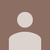0

# Shortest distance between two models

SGU 6 years ago updated by ไอยดา สุรีวงค์ 2 years ago

Hello,

Is there a way to get the shortest distance between two Model3D objects in a ViewPort3D?

I found Method GetDistanceSquared(Point3D, Visual3D) and it partially do the job if I assign my Model3D to a Visual3D, but I don't have a Point as a reference but another Model3D.

//This is what I used to get the distance Point-Model

ModelVisual3D model = new ModelVisual3D();
model.Content = axis1;  //where axis1 is a Model3D
Distance.Content = Math.Sqrt(ElementSortingHelper.GetDistanceSquared(new Point3D(0, 0, 0), model));

I was thinking that I could get the shortest distance in between all the points from the first model compared with the second model but maybe there is a faster or simpler way to do it with a Method that I'm missing.

Thanks a lot.